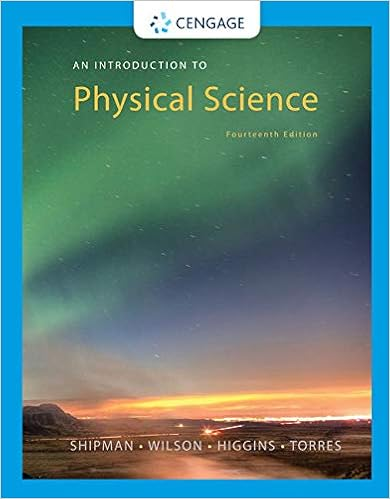# 50 ma 100 ma 150 ma magnetic polarity time scale part

• 14
• 95% (22) 21 out of 22 people found this document helpful

This preview shows page 1 - 4 out of 14 pages.

##### We have textbook solutions for you!
The document you are viewing contains questions related to this textbook.The document you are viewing contains questions related to this textbook.
Chapter 21 / Exercise 1
An Introduction to Physical Science
Shipman/WilsonExpert Verified
50 Ma100 Ma150 MaMagnetic PolarityTime ScalePart 1: Analysis of the Present State of Geoworld
##### We have textbook solutions for you!
The document you are viewing contains questions related to this textbook.The document you are viewing contains questions related to this textbook.
Chapter 21 / Exercise 1
An Introduction to Physical Science
Shipman/WilsonExpert Verified
2 “Geoworld” Plate Tectonics Lab 1. On the map on page 9, highlight the mid-ocean ridge in the Elrond Sea and label it the Hobbit Ridge. 2. Draw a diagram and explainthe origin of the striped magnetic anomaly pattern visible in the Elrond Sea.3. The pattern of positive and negative magnetic anomalies in the Elrond Sea can be correlated with the pattern of normal and reversed magnetism on the magnetic polarity time scale. Based on this correlation, how many million years (m.y.) ago did sea floor spreading begin in the Elrond Sea?
4. Using the magnetic anomaly pattern in the Elrond sea, determine the 1/2-spreading rate on the Hobbit Ridge (i.e., determine how fast the Frodo continent is moving away from the Hobbit ridge). Give your answer in mm/y (millimeters per year). Show your equations and explain where each number came from.
rate
5. What is the full spreading rate (in mm/y) of the Hobbit Ridge? (i.e., how fast are the Bilbo and Frodo continents moving away from each other)? Show your equations and explain where each number came from.
“Geoworld” Plate Tectonics Lab 3 6. Using the magnetic anomaly patterns in the Aragorn Ocean, determine when sea floor spreading started to form the Aragorn Ocean crust (remember, spreading is not actively going on now but it was at one time). Spreading Started __ m.y. ago 7. At the time that active sea-floor spreading was occuring in the Aragorn Ocean, at what rate was new sea-floor being created? (Think: is this related to the full spreading rate or the half spreading rate)? Show your equations and explain where each number came from.
B. Plate Boundaries 1. There are two plate boundaries on the map. You already highlighted one of them in Part 1, A. 1. above. Highlight the other boundary also.2. Give one example of each of the following types of plate boundaries on Geoworld (Note: the boundary between two plates can vary in type from place to place).
3. How many lithospheric plates are there in all?C. Cross-Section Make a schematic cross-section of Geoworld along A-A'. Draw it in the box provided at the bottom of the map on page 9. Show the entire thickness of the lithosphere and part of the asthenosphere. Label the crust, mantle lithosphere and asthenosphere.
•••